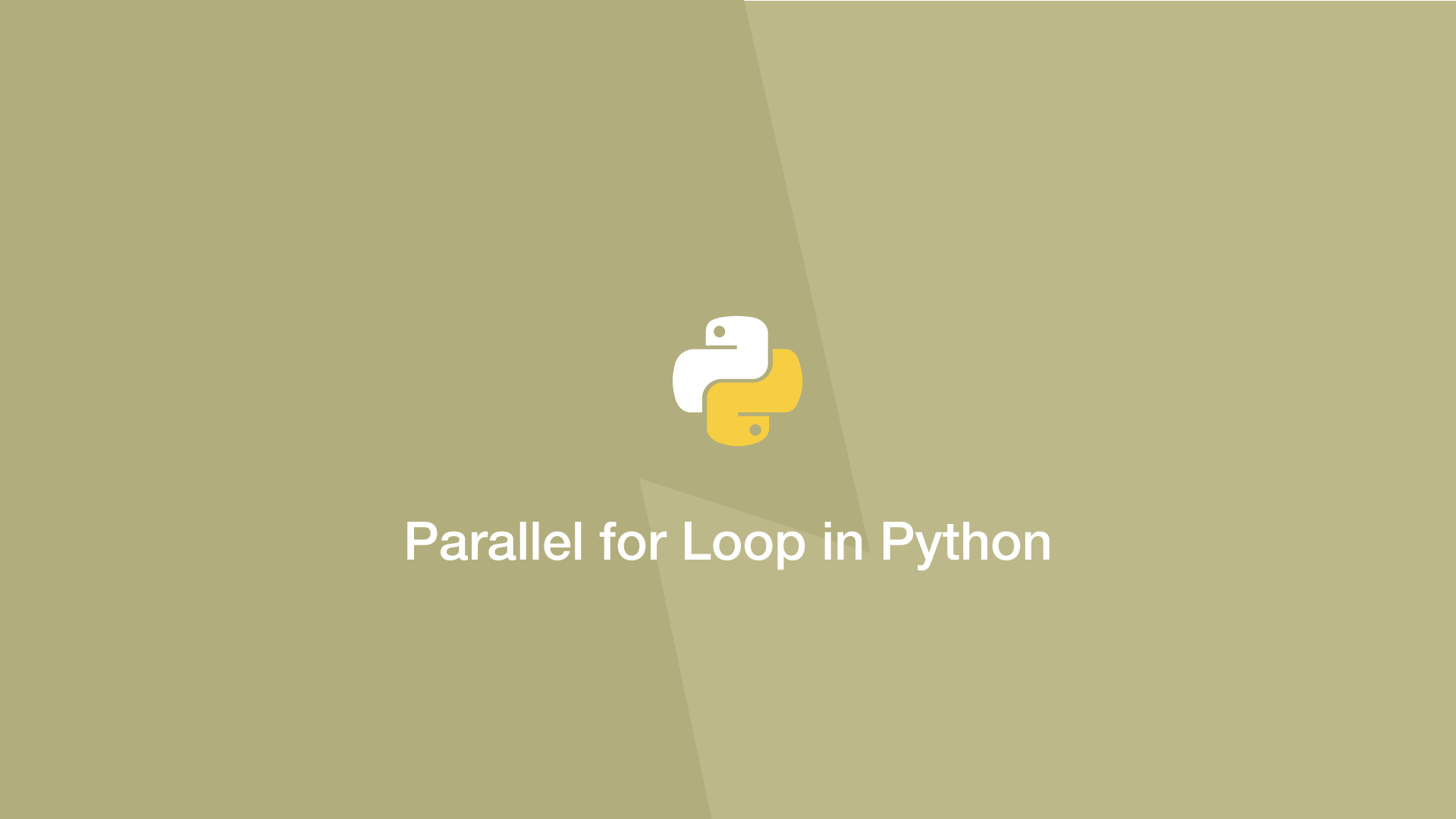# Parallel for Loop in PythonParallelizing a for loop in Python means spreading the process over all the cores on a multicore system. Each loop can run in parallel on a different core, speeding up the overall processing time.

This tutorial will cover how to run for loops in parallel in Python.

## Parallelize for Loop in Python Using the multiprocesssing Package

To parallelize a loop we can use the `.Pool()` object from the multiprocessing package. The example below demonstrates looping over a function 10 times using a `multiprocessing.Pool()` object.

``````import multiprocessing

def multiply(v):
return v * 10

pool_obj = multiprocessing.Pool()

print(pool_obj)

res = pool_obj.map(multiply,range(0,10))
``````

## Use the joblib Package to Parallelize for Loop in Python

Here is another example using the `joblib` package to call a function 10 times:

``````from joblib import Parallel, delayed
def process(i):
return i * i

results = Parallel(n_jobs=2)(delayed(process)(i) for i in range(10))
``````

#### Related Tutorials### How to Use For Loops in Python

September 02, 2020### How to Use While Loops in Python

September 03, 2020### How to Use Python List Comprehension

October 04, 2020### How to Read a File Line by Line in Python

September 21, 2020### The Python Sleep Function

October 01, 2020### How to Enumerate in Python

September 17, 2020